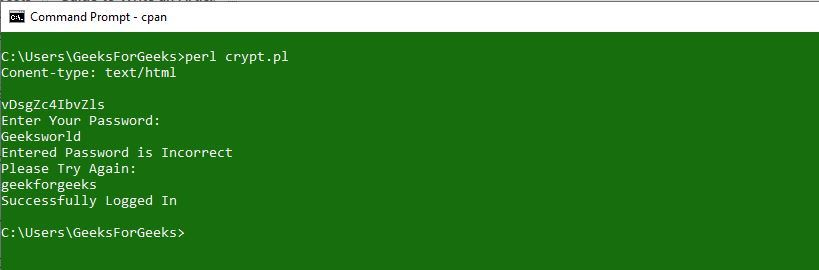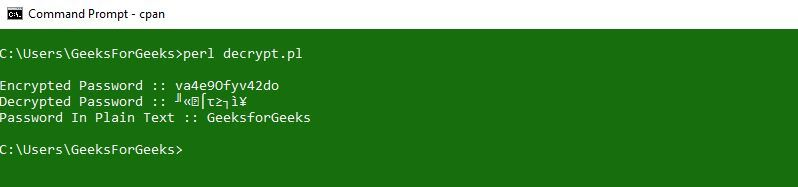# Encryption and Decryption In Perl

Crypt function in, Perl, is basically used to store sensitive data and passwords using ASCII characters as encrypted strings (this function encrypts the string). Strings can only be encrypted, they can not be decrypted in the same way as encryption is done.

Syntax: \$encyrpted_string = crypt \$string, \$salt;

Arguments passed to the function:

• \$string: it is the string that needs to be encrypted.
• \$salt: used for selecting an encrypted version from different variations.

Return Value: Function returns an encrypted string

Note: `\$salt` variable can be the combination of any two characters from the below given set:

`['.', '/', 0..9, 'A'..'Z', 'a'..'z']`

We can use/include more characters other than this given set of characters, this set is just used for the purpose of recommendation. First two characters in the encrypted string are stored as the salt character which can be used for later comparisons. We can even select the characters for salt by using rand function(random selection). We can observe /see large changes in the resulting / final encrypted string, if small changes are made in the \$string or \$salt.

Example: Below is the example to illustrate the above mentioned crypt function for Encryption.

 `#!usr/bin/perl ` `print` `"Conent-type: text/html\n\n"``; ` ` `  `# Setting the password ` `\$password` `= ``'geekforgeeks'``; ` ` `  `# Encrypting the password using crypt function ` `\$hidden` `= ``crypt` `\$password``, ``join` `""``,  ` `          ``(``'.'``, ``'/'``, 0..9, ``'A'``..``'Z'``, ``'a'``..``'z'``) [``rand` `64, ``rand` `64]; ` ` `  `print` `"\$hidden \n"``; ` ` `  `\$salt` `= ``substr` `(``\$hidden``, 0, 2); ` ` `  `# Taking user input ` `print` `"Enter Your Password: \n"``; ` `while` `() ` `{ ` `    ``if` `(``\$hidden` `eq (``crypt` `\$_``, ``\$salt``)) ` `    ``{ ` `        ``print` `"Successfully Logged In \n"``; ` `        ``exit``; ` `    ``} ` `    ``else`  `    ``{ ` `        ``print` `"Entered Password is Incorrect \n"``; ` `        ``print` `"Please Try Again: \n"``; ` `    ``} ` `} `

Output:### Decryption In Perl

For decryption, the encrypted password in Perl needs to be decrypted using the `MIME::Base64` module. For decrypting a string we can call or use `decode_base64()` function. A single argument in the form of the string is taken as the input by the function in order to return the decoded or decrypted data/password.
Syntax:

Use MIME::Base64;
\$decoded = decode_base64();

Example: Given below is the example that illustrate the decryption process in Perl.

 `#!/usr/bin/perl ` `use` `strict; ` `use` `warnings; ` `use` `MIME::Base64; ` ` `  `# Setting the password ` `my` `\$password` `= ``"GeeksforGeeks"``; ` ` `  `# For encrypting the plaintext password  ` `# using crypt function ` `my` `\$encoded` `= ``crypt` `\$password``, ``join` `""``,  ` `             ``(``'.'``, ``'/'``, 0..9, ``'A'``..``'Z'``, ``'a'``..``'z'``) [``rand` `64, ``rand` `64]; ` ` `  `my` `\$salt` `= ``substr` `(``\$encoded``, 0, 2); ` ` `  `# For decrypting the encrypted password ` `# using base_64 module  ` `my` `\$decoded` `= decode_base64(``\$encoded``); ` `print` `"\n"``; ` ` `  `# For printing the Encrypted password ` `print` `"Encrypted Password :: \$encoded\n"``; ` ` `  `# For printing the Decrypted password ` `print` `"Decrypted Password :: \$decoded\n"``; ` ` `  `# For printing the password in PlainText  ` `print` `"Password In Plain Text :: \$password\n"``; `

Output:My Personal Notes arrow_drop_upCheck out this Author's contributed articles.

If you like GeeksforGeeks and would like to contribute, you can also write an article using contribute.geeksforgeeks.org or mail your article to contribute@geeksforgeeks.org. See your article appearing on the GeeksforGeeks main page and help other Geeks.

Please Improve this article if you find anything incorrect by clicking on the "Improve Article" button below.

Article Tags :
Practice Tags :

Be the First to upvote.

Please write to us at contribute@geeksforgeeks.org to report any issue with the above content.